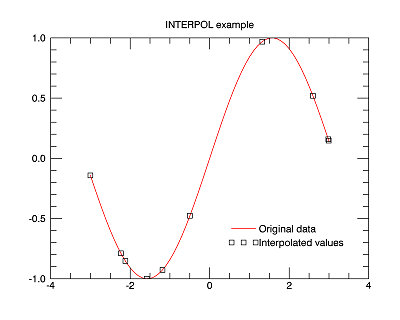The INTERPOL function performs linear, quadratic, or spline interpolation on vectors with a regular or irregular grid.

Note: The INTERPOL function automatically detects any NaN values in your input data, and excludes these values when computing the interpolation.

This routine is written in the IDL language. Its source code can be found in the file interpol.pro in the lib subdirectory of the IDL distribution.

## ExamplesCreate a floating-point vector of 61 elements in the range [-3, 3].

`; Make a sine wave on the interval [-3,3]`
`x = FINDGEN(61)/10 - 3`
`y = SIN(x)`
` `
`; Define a set of 10 locations where interpolates are desired.`
`xinterp = RANDOMU(1, 10)*6 - 3`
` `
`; Interpolate.`
`result = INTERPOL(y, x, xinterp)`
` `
`; Plot the original sine wave and the interpolated values.`
`p = PLOT(x, y, COLOR='red', NAME='Original data', \$`
`   TITLE='INTERPOL example')`
`q = SCATTERPLOT(xinterp, result, SYMBOL='square', /OVERPLOT, \$`
`   NAME='Interpolated values')`
`lgd = LEGEND(POSITION=[3.5,-0.5], /DATA, SHADOW=0, LINESTYLE='none')`

## Syntax

For regular grids:

Result = INTERPOL( V, N [, /LSQUADRATIC] [, /NAN] [, /QUADRATIC] [, /SPLINE] )

For irregular grids:

Result = INTERPOL( V, X, XOUT [, /LSQUADRATIC] [, /NAN] [, /QUADRATIC] [, /SPLINE] )

## Return Value

The result is a single- or double-precision floating-point vector, or a complex vector if the input vector is complex.

## Arguments

### V

An input vector of any type except string.

### N

The number of points in the result when both input and output grids are regular. The abscissa values for the output grid will contain the same endpoints as the input.

### X

The abscissa values for V, in the irregularly-gridded case. X must have the same number of elements as V, and the values must be strictly ascending or descending.

### XOUT

The abscissa values for the result. The result will have the same number of elements as XOUT. XOUT does not need to be monotonic.

## Keywords

If set, interpolate using a least squares quadratic fit to the equation y = a + bx + cx2, for each 4 point neighborhood (x[i-1], x[i], x[i+1], x[i+2]) surrounding the interval of the interpolate, x[i] ≤ XOUT < x[i+1].

If set, interpolate by fitting a quadratic y = a + bx + cx2, to the three point neighborhood (x[i-1], x[i], x[i+1]) surrounding the interval x[i] ≤ XOUT < x[i+1].

### NAN

Set this keyword to filter out NaN values before interpolating. The default behavior is to include the NaN values - by including NaN's the output will contain NaN's in locations where the interpolation result is undefined.

### SPLINE

If set, interpolate by fitting a cubic spline to the 4 point neighborhood (x[i-1], x[i], x[i+1], x[i+2]) surrounding the interval, x[i] ≤ XOUT < x[i+1].

Note: If LSQUADRATIC or QUADRATIC or SPLINE is not set, the default is to use linear interpolation.

## Version History

 Original Introduced 7.1 Renamed the U argument to XOUT. 8.0 Added NAN keyword.